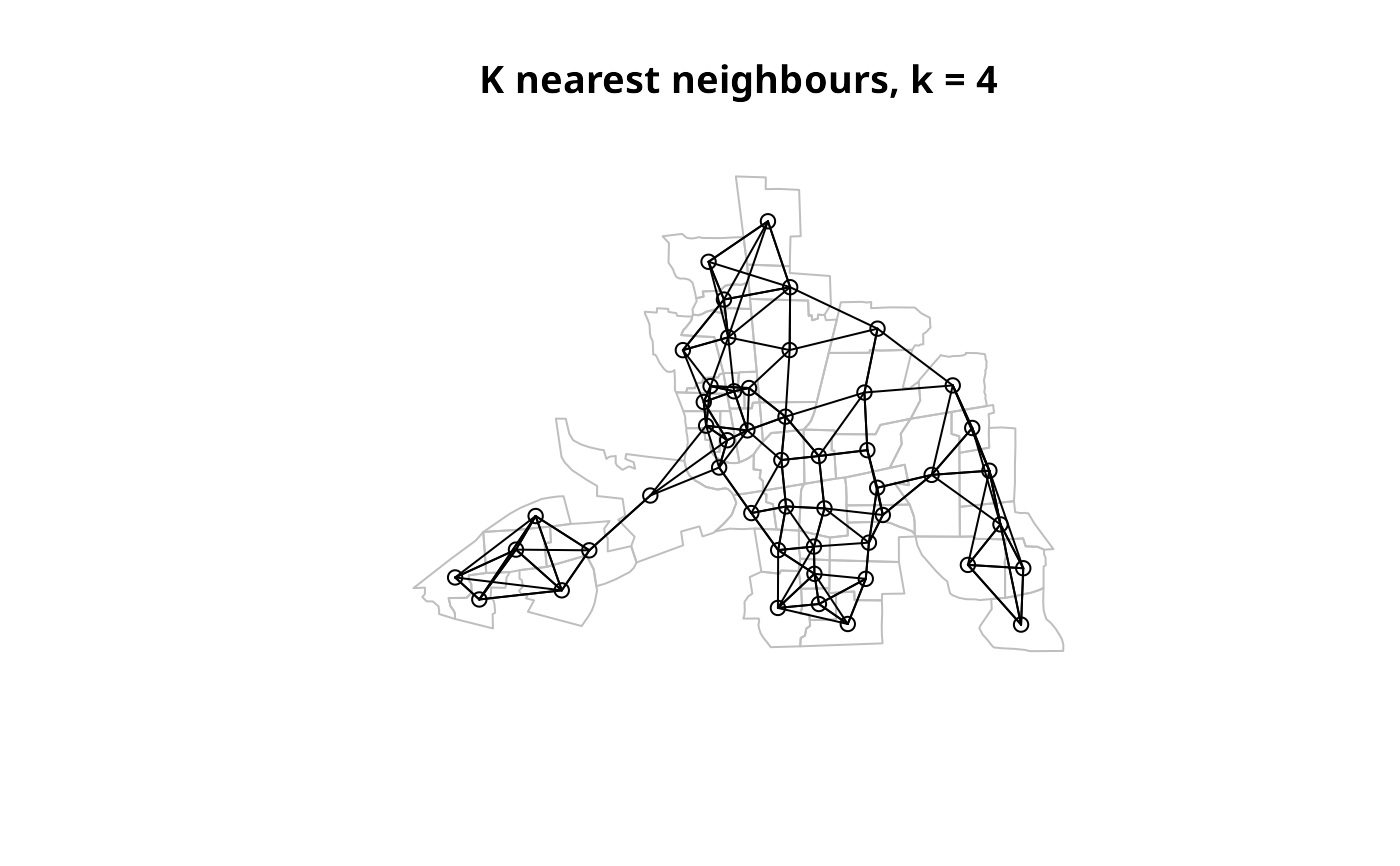The function converts a knn object returned by knearneigh into a neighbours list of class nb with a list of integer vectors containing neighbour region number ids.

knn2nb(knn, row.names = NULL, sym = FALSE)

## Arguments

knn

A knn object returned by knearneigh

row.names

character vector of region ids to be added to the neighbours list as attribute region.id, default seq(1, nrow(x))

sym

force the output neighbours list to symmetry

## Value

The function returns an object of class nb with a list of integer vectors containing neighbour region number ids. See card for details of “nb” objects.

## Author

Roger Bivand Roger.Bivand@nhh.no

knearneigh, card

## Examples

columbus <- st_read(system.file("shapes/columbus.shp", package="spData"), quiet=TRUE)
coords <- coordinates(as(columbus, "Spatial"))
col.knn <- knearneigh(coords, k=4)
plot(st_geometry(columbus), border="grey")
title(main="K nearest neighbours, k = 4")